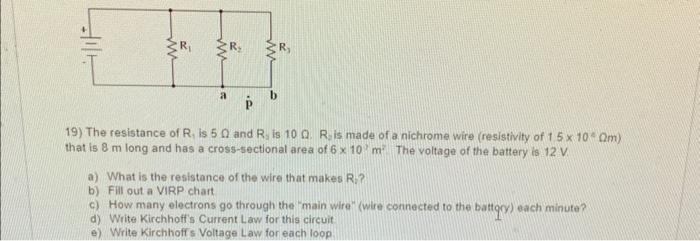# (Solved): a-e please 19) The resistance of R1 is 5 and R3 is 10. R2 is made of a nichrome wire (r ...19) The resistance of is and is . is made of a nichrome wire (resistivity of ) that is long and has a cross-sectional area of The voltage of the battery is . a) What is the resistance of the wire that makes R:? b) Fill out a VIRP.chart c) How many electrons go through the "main wire" (wire connected to the baftory) each minute? d) Write Kirchhoff's Current Law for this circuit e) Write Kirchhoff s Voltage Law for each loop

We have an Answer from Expert

Given: The resistance of R1 is 5 ? and R2 is 10 ? R2 is made of nichrome wire ( resistivity of 1.5 * 10 ^(-2)

?m that is 8 m long and has a cross-sectional area of (6 x 10^(-6) m^2). The voltage of the battery is 12V

a) To find the resistance of the wire that makes R2:

Here To find the resistance of the wire that makes R, we need to use the formula:

Resistance = (resistivity x length) / area

Resistance of R2 = (1.5 x 10^(-2) ?m x 8m) / (6 x 10^(-6) m^2)
Resistance of R2 = 200 ?

So the total resistance of the circuit is:

R = R1 + R2 = 5? + 200? = 205?

We have an Answer from Expert